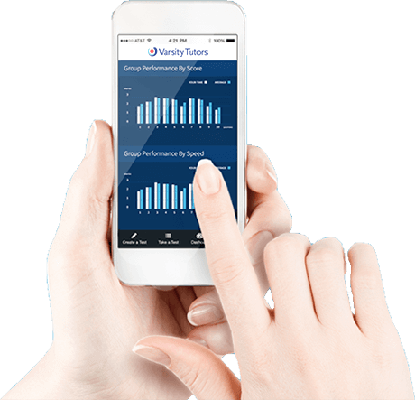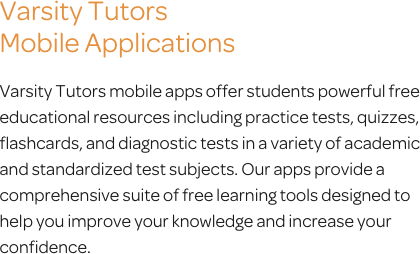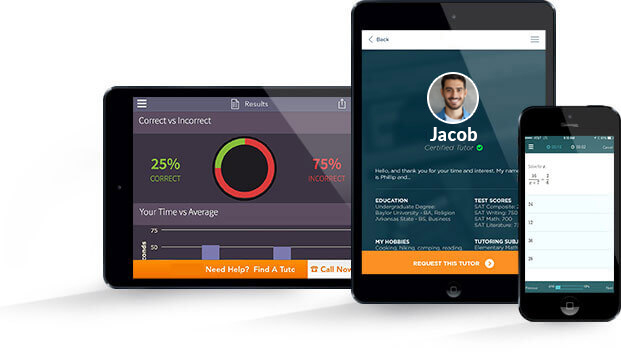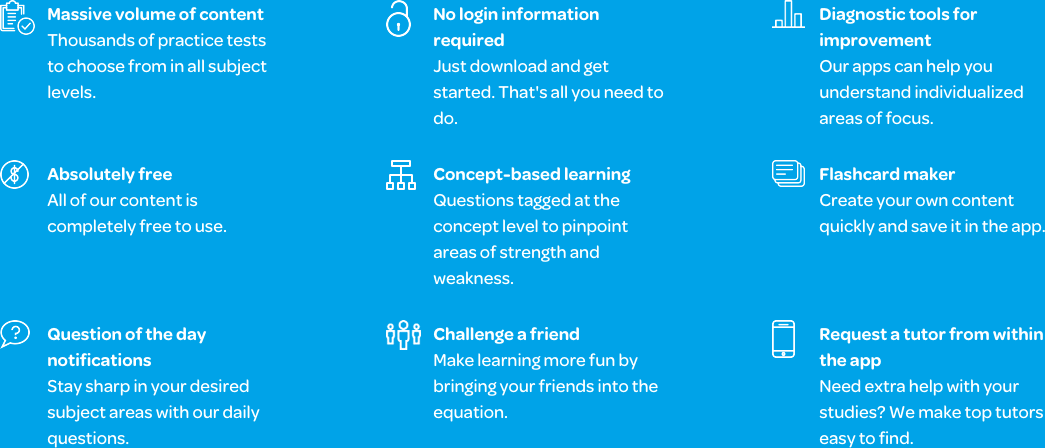# Varsity Tutors Calculus 1 mobile app### The Varsity Tutors Calculus 1 Mobile App

Calculus 1 involves working with functions-- those that are odd or even, increasing or decreasing, and those of different types. In the course, you are sure to encounter polynomials; trigonometric functions; and rational, exponential, and logarithmic functions. Limits and derivatives will fill your time, and possibly test your patience, as well. The Varsity Tutors Calculus 1 app for iPad, iPhone, and Android devices offers several tools to aid in studying for the subject. The tools, which include Full-Length Practice Tests, shorter topic-specific practice tests, flashcards, and more, are designed based on the relevant content you are learning in class, therefore effectively complementing your studies.

The Full-Length Practice Tests you’ll find in the app offer several benefits. Questions are in series and in random order, so you can get accustomed to the randomness and calling on your math skills under pressure. Also, you can quickly see the concepts that come more natural to you and those you need more work on. Evaluations at the end of each practice test tell how you did compared to other test takers and track your accuracy and speed. Explanations at the end of each evaluation show why you answered incorrectly, and they can also be used to enhance your skills even if the question was answered correctly. These tools can help you customize your study plan, allowing you to make the most of your study time.

The Calculus 1 app offers even more resources to aid in your study sessions, including shorter concept-based tests, which focus on individual topics you can choose from. In addition to the hundreds of practice exercises, there is also a Question of the Day. This tool picks a random question from the database of items, so you can get a fast review each day, yet not know exactly what that will be. The Learn by Concept interactive syllabus enables you to select specific concepts and sub-topics as well. For each, you get a series of questions, answers, and study content to help guide you along. The flashcards let you do this as well, but you complete one question at a time and get immediate feedback on each. You can do as many or as few as you want, and even customize the pre-made items with text, audio, and video on your mobile device.

Together, these tools will enable you to review, study, and comprehend the topics of Calculus 1. You will get relevant and ample practice on limits, continuity, and derivatives. From the Power Rule, Product Rule, Quotient Rule, or Chain Rule, to applying rates of change and trigonometric derivatives, the app allows any prospective test taker to maximize their chances of success in the course. Calculus 1 moves fast – soon you move on to logarithmic and exponential function derivatives and hyperbolic trigonometric function derivatives. You’ll move from simple integrals to Riemann sums and the Fundamental Theorem of Calculus. You may even get an intro to differential equations.

Calculus 1 is complex, and it acts as an introduction to even more complex mathematics. The Varsity Tutors Calculus 1 app for iOS and Android, available on iTunes and the Google Play Store, can give you just the practice you need to master the concepts covered in your class.Calculus 1 is an introduction to the field of calculus. The word calculus comes from the Latin word, which literally means a small pebble used for counting. Calculus is the mathematical study of change. The two major branches of calculus are differential calculus and integral calculus, which are related to one another through the fundamental theorem of calculus. Differential calculus is concerned with slopes and the rates of change of curves. Integral calculus is focused on the accumulation of quantities and the areas between and under curves. It often comes as a surprise to students how such an abstract concept is able to describe and explain the world around them. It can be used to better understand social, biological, and physical sciences. Calculus 1 is suitable for those students with a firm understanding of algebra and geometry, even if they have never before been exposed to calculus concepts. While Calculus 1 can be a big leap in mathematical conceptualization, you can get extra help with the free Varsity Tutors Calculus 1 app for iPhone, iPad, and Android.

You will be introduced to both differential and integral calculus in your Calculus 1 course. Concepts from algebra and geometry will be built upon and may include limits, derivatives, application of derivatives, and integrals. Additionally, you will be introduced to various calculus functions, differential equations, and spatial calculus.

There is not a single standardized exam for Calculus 1. Instead, the professor will typically monitor student progress in specific concepts over the course of the class with periodic quizzes and tests. Cumulative exams are often given at the midpoint and end of the course. The course syllabus will usually outline the order the topics that will be covered and how they will be weighted for the final grade. It is essential that students master each of the concepts before moving on to more advanced concepts, because they are directly related to later concepts. Many of the concepts are intrinsically bound together, and it is impossible to understand later concepts such as integrals without first understanding the rules and methods that apply to derivatives. Because of this, students should pay close attention to the grades and feedback they receive throughout the course as a guide for where they need to focus further attention and study efforts. Additionally, you can get comprehensive coverage of all likely Calc 1 topics when you download the Varsity Tutors Calculus 1 app from iTunes or the Google Play Store.

During this course, you will learn to define a limit and a limit notation. Students will learn to find the limit of a function graphically. This helps give a broader understanding of the limit, how the limit is found, and why limits are used. Various graphs are used to find one and two-sided limits. Limit rules allow students to evaluate a limit algebraically without a graph. Indeterminate Form is introduced to allow students to evaluate a limit function when the result is infinity divided by infinity or zero divided by zero. When this occurs, it is necessary to simplify the function to find the answer. These skills are critical in finding derivatives and integrals later in the course.

Infinity is a complicated concept, and may seem counterintuitive in relation to limits. To understand limits approaching infinity, it is first necessary to understand the concept of infinity. Then how that concept applies to the study of indeterminate forms and end behavior. The key to this concept is understanding that one cannot solve for an infinite endpoint, as that point cannot be known. Instead, it is necessary to find a point approaching infinity and solve for that. Other concepts that will be covered in relation to limits include the Epsilon Delta definition, limits and continuity, and derivatives as they relate to limits.

Within the study of limits, you will learn how to calculate the instantaneous velocity, or average rate of change, of a function at a specific point. This particular limit is so important that it receives its own name: a derivative. There are four basic rules that apply to derivatives; the Power Rule, Product Rule, Quotient Rule, and Chain Rule. The Power Rule can be used for all polynomials, and allows you to find the slope of a function at any given point. The Product Rule allows you to find the derivative of a function that is expressed as the product of two or more functions. The Quotient Rule is a formula to divide one function by another, and it is essential to understanding logarithmic properties. The Chain Rule explains differentiation composition functions, which simply means it gives you a way to apply one function to the results of a different function. You will also study derivatives of exponential and logarithmic functions, trigonometric derivatives, inverse trigonometric derivatives, inverse function derivatives, and higher order derivatives. Sound like a lot? You can drill down into this and all other topics from any location when you download the Varsity Tutors Calculus 1 app from iTunes or the Google Play Store.

There are numerous applications of derivatives, including understanding particle motion, finding velocity and acceleration, finding when an object is at rest, finding when an object changes direction, finding speed, and graphing derivatives with particle motion. Some of the theorems that illustrate these applications include the Intermediate Value Theorem, Mean Value Theorem, Rolle’s Theorem, and Absolute Extrema. You will learn to find an approximation of rate, the constant of proportionality of rate, the differentiable of rate, the rate of change, to create prediction models, find the rate of flow, and to solve for time. Calculus also plays an important role in spatial reasoning. Spatial calculus can be used to find acceleration, distance, position, and velocity.

Integrals are another important component of calculus. An integral is a mathematical object that can be interpreted as a generalized or specific area. Specifically, it is the process of finding the area beneath a curve. To accomplish this, you will learn to calculate Riemann Sums. Building upon this, integration rules are simply the reverse of differentiation rules. Similarly, finding exponential, logarithmic, and trigonometric integrals require the opposite function; instead of multiplying, you divide.

At the end of this course, students will have a thorough foundation of the principles of calculus and be prepared for the more advanced topics of Calculus 2 and Calculus 3. There are many fields of study that may require a student to master calculus concepts, including engineering, science, and medicine. Whatever your pursuits, you can help yourself prepare for future success with the comprehensive study tools available in Varsity Tutors’ Calculus 1 app, a free download for your iPad, iPhone, or Android-powered device. Download it today and get started.